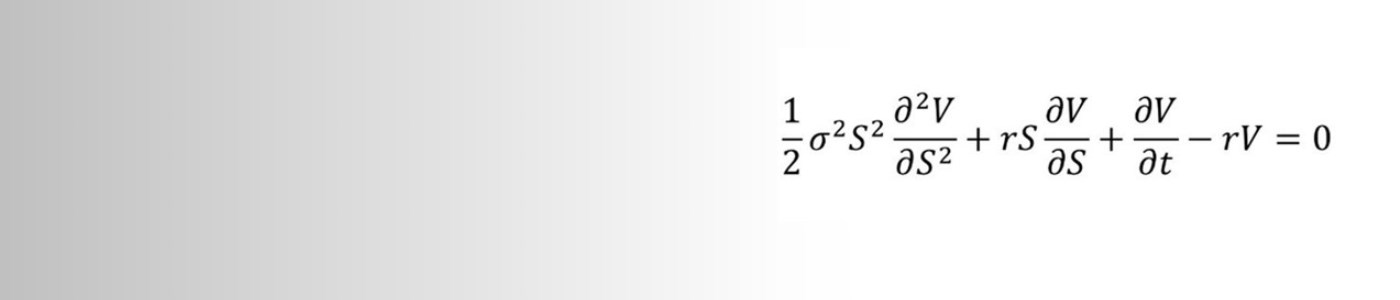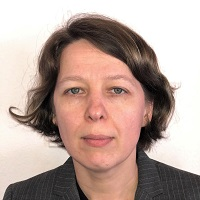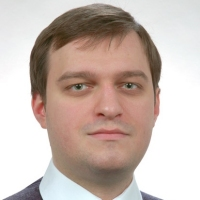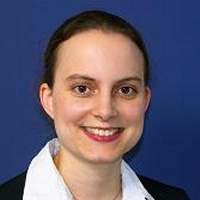# Financial Mathematics and Control Theory Research

The area of financial mathematics is concerned with the development and the analysis of models that can be of use to the valuation of investments in financial assets. Since the pioneering days of Black and Scholes, the area has attracted increasingly interest, reflecting the growth in the business of financial institutions. The part of financial mathematics that is concerned with the valuation of investment decision strategies overlaps with the theory of control and optimisation, which is a traditional branch of mathematics with a wide and far-reaching range of applications. Developments in both areas involve advanced theory from several areas of mathematics, including probability and stochastic processes, analysis, and partial differential equations.

*Note to prospective PhD candidates ONLY: please do not contact Financial Mathematics and Control Theory academics directly regarding PhD entry.  Instead, please refer to MPhil and PhD in Mathematics.*

Individual faculty interests are listed below, along with our Research students.

## Dr Christoph Czichowsky• Financial mathematics
• Stochastic optimal control
• Stochastic analysis
• Optimal portfolio choice
• Market frictions
• Transaction costs
• Duality
• Mean-variance portfolio optimisation

## Dr Albina Danilova• Stochastic calculus and financial mathematics, in particular: filtering, enlargement of filtrations and stochastic control and optimisation
• Derivatives pricing and hedging in incomplete markets and/or under asymmetric information
• Utility maximization and equilibrium

## Dr Pavel Gapeev• Stochastic calculus
• Optimal stopping and free-boundary problems
• Pricing of American options
• Sequential testing and disorder detection problems
• Interest rate and credit risk models
• Illiquidity markets
• Stochastic impulse control and optimisation
• Gaussian processes• Probability and financial mathematics, with special emphasis on hedging and pricing of derivatives
• Utility maximization and equilibrium
• Real investment decisions under uncertainty.• Mathematical finance, with a particular interest in real options and accounting theory, including Corporate Disclosure policy and Bargaining Theory
• Set-theoretic and general topology
• Topics in analysis such as automatic continuity and regular variation

Professor Johannes Ruf• Financial Mathematics
• Stochastic Portfolio Theory
• Stochastic Analysis
• Applied Probability
• Econometrics
• Economic Learning Models
• Green Finance

## Professor Amol Sasane• Applicable analysis, in particular control theoretic problems for models described by partial differential equations
• Systems of partial differential equations
• Algebras of holomorphic functions

## Professor Luitgard Veraart• Financial mathematics
• Statistics in finance
• Risk management in financial markets
• Systemic risk
• Networks
• Modelling of energy markets
• Optimal investment problems
• Stochastic volatility models

## Professor Mihail Zervos• Stochastic analysis
• Stochastic control and optimisation
• Optimal stopping problems
• Valuation of investment decisions and investments in real options
• Options of American type
• Derivative pricing in incomplete markets
• Weather derivatives